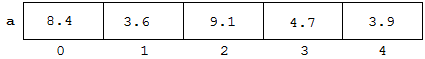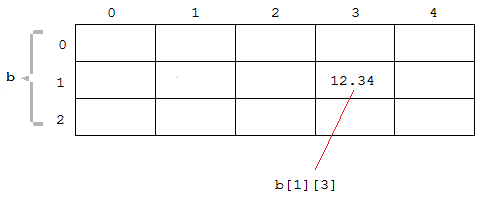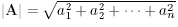http://cpp.gantep.edu.tr
C++ resources. Faculty of Engineering, University of Gaziantep
MAIN  -  Tutorials  -  Howtos  -  nmPrimer  -  Misc  -  Links  -  Contacts
C++ Tutorial (Basic)

7. Arrays

Complete: ##################-- (90%)

7.1 Introduction
7.2 Array Declarations
7.3 Initializing Arrays
7.4 Multidimensional Arrays
7.5 Passing Arrays to the Functions
7.6 Examples
7.7 Exercises

7.1 Introduction

An array is a group of objects (such as variables or constants), all of the same name and data type. The objects are called the elements of the array and are indexed consecutively as 0, 1, 2, 3, .... These numbers are called indices of subscripts of the array. After declaration of an array, the elements are stored in an order one after the other in the computer memory location where they exist during the program execution.

In this section we will consider how to declare and use arrays in C++.

7.2 Array Declarations

The declaration of an array is similar to that of an ordinary variable but with the addition of a declaration of the number of elements in the array:
```  data-type array-name[numberOfElements];
```

For example,

```  int mass; // 10-element int array, with indices 0,1,2,3,4,5,6,7,8,9
double a;  // 5-element double (real) array, with indices 0,1,2,3,4
```
The 5 elements of the array can be assigned as follows:
```  double a;
...
a = 8.4; // first element
a = 3.6;
a = 9.1;
a = 4.7;
a = 3.9; // last element
```
In memory this array looks like in Fig. 7.1.Fig 7.1: Elements and corresponding indices of the 5-element double array a in memory.

 Note that The array index starts from 0, not from 1. For the array a, the index of the first element is 0 and the last element is 4. Element a accesses a memory location that is outside the array and so using this element will probably lead to unexpected results!

Program 7.1 inputs 5 numbers, assigns them to an array, and then outputs them in reverse order.

07prg01.cpp: Assigning and outputting an array
 ``` 1: 2: 3: 4: 5: 6: 7: 8: 9: 10: 11: 12: 13: 14: 15: 16: 17: 18: 19: ``` ```// Assigning and outputting an array #include using namespace std; int main () { double a; cout << "Input 5 real numbers:" << endl; for(int i=0; i<5; i++) cin >> a[i]; cout << "The reverse order: " << endl; for(int i=4; i>=0; i--) cout << a[i] << ' '; cout << endl; return 0; }``` ```Input 5 real numbers: 1.2 3.5 -0.4 10.2 7.1 The reverse order: 7.1 10.2 -0.4 3.5 1.2 ```

The important rule in the declaration of an array is: numberOfElements must be a positive integer constant. A declaration of a compile-time array of the form:
```  const int n = 10;
float x[n];
```
causes the compiler to allocate a block of memory large enough to hold 10 float values.

Defined (symbolic) constants are also allowed:

``` #define N  10
.
.
float x[N];
```
However, C++ does not allow us to write:
```  int n;
cout << "how many elements? ";
cin >> n;
float x[n]; // *** not allowed at run-time! ***
```
That is, at run-time the user can not determine the size of an array, since arrays are blocks of non-dynamic memory. To obtain dynamic sized arrays we can use dynamic arrays. Details of the dynamic memory management are described in Advanced Tutorial, Section 2.

In Program 7.2, Program 7.1 is modified such that it uses the const keyword to define the size of the array.

07prg02.cpp: Reading and outputting an array (modified)
 ``` 1: 2: 3: 4: 5: 6: 7: 8: 9: 10: 11: 12: 13: 14: 15: 16: 17: 18: 19: 20: ``` ```// Assigning and outputting an array (modified) #include using namespace std; int main () { const int n = 5; double a[n]; cout << "Input " << n << " real numbers:" << endl; for(int i=0; i> a[i]; cout << "The reverse order: " << endl; for(int i=n-1; i>=0; i--) cout << a[i] << ' '; cout << endl; return 0; }``` ```Input 5 real numbers: 1.2 3.5 -0.4 10.2 7.1 The reverse order: 7.1 10.2 -0.4 3.5 1.2 ```

At run time, you can get the size of an array via the sizeof() operator as follows:

```  float x;
.
.
int n = sizeof(x);                   // the size of the array in the memory (10*4 = 40 bytes for a 32 bit machine)
int size = sizeof(x)/sizeof(float);  // number of elements 40/4 = 10
```

7.3 Initializing Arrays

An array can be initialized with a single initializer list, like this:
```  double a = {8.4, 3.6, 9.1, 4.7, 3.9};
```
If the array has more elements than values listed, then the remaining elements are initialized to zero. Hence,
```  double a = {8.4, 3.6};
```
is the shorthand for
```  double a = {8.4, 3.6, 0.0, 0.0, 0.0};
```
Program 7.3 shows how to initialize arrays explicitly.

07prg03.cpp: Initializing array
 ``` 1: 2: 3: 4: 5: 6: 7: 8: 9: 10: 11: 12: 13: 14: 15: 16: 17: 18: 19: 20: 21: 22: 23: 24: ``` ```// Initilising an array #include using namespace std; int main () { const int n = 5; double a[n] = {8.4, 3.6, 9.1, 4.7, 3.9}; double b[n] = {-1.0, 1.0}; double c[n] = {0.0}; for(int i=0; i

The use of the size specifier (numberOfElements) may be omitted. For example,
```  double a[] = {8.4, 3.6, 9.1, 4.7, 3.9};
```
is equivalent to the declaration,
```  double a = {8.4, 3.6, 9.1, 4.7, 3.9};
```

7.4 Multidimensional Arrays

The arrays considered so far are known as one-dimensional arrays. The elements of an array can be almost any type, including an array type - an array of arrays is called a multidimensional array, e.g. a one-dimensional array of one-dimensional arrays is a two dimensional array.

The following declarations are valid

```  double a;       // 5-element one-dimensional array
float b;     // 15-element two-dimensional array
int c;   // 200-element three-dimensional array
```
The statement
```  b = 12.34;
```
assigns the value 12.34 to the element identified by (1,3), and can be visualised as in Fig 7.2.Fig 7.2: Elements and corresponding indices of two-dimensional array b.

Note that, one-dimensional and two-dimensional arrays are known as vectors and matrices, respectively.

Program 7.4 reads the elements of two matrices and outputs the sum matrix, C = A + B, according to matrix addition rule.

07prg04.cpp: Using two dimensional arrays
 ``` 1: 2: 3: 4: 5: 6: 7: 8: 9: 10: 11: 12: 13: 14: 15: 16: 17: 18: 19: 20: 21: 22: 23: 24: 25: 26: 27: 28: ``` ```// Using two-dimensional arrays #include using namespace std; int main () { const int COL = 3, ROW = 2; double a[ROW][COL], b[ROW][COL], c[ROW][COL]; cout << "Input matrix A:\n"; for(int i=0; i> a[i][j]; cout << "Input matrix B:\n"; for(int i=0; i> b[i][j]; cout << "Matrix C = A+B:\n"; for(int i=0; i

7.5 Passing Arrays to the Functions

Arrays can be passed to the functions in similar way as an ordinary variable passed by reference. In C++ this is acheived by passing the memory address of the first element of the array (denoted by a[]) and an additional integer n that states the number of elements of the array. The function can then access the array elements using a index in the range 0 and n-1.

This is illustrated below in Program 7.5 where the function sum() returns the sum of the values in array a. Note that double x[] in the function declaration indicates to the compiler the memory address and type of the array, but not the size of the array.

07prg05.cpp: Passing an array to a function
 ``` 1: 2: 3: 4: 5: 6: 7: 8: 9: 10: 11: 12: 13: 14: 15: 16: 17: 18: 19: 20: 21: 22: 23: 24: 25: 26: 27: 28: 29: ``` ```// Passing an array to a function #include using namespace std; double sum(double x[], int); int main () { double a; cout << "Enter 5 reals: "; for (int k=0; k<5; k++) cin >> a[k]; double s = sum(a, 5); cout << "The sum is " << s << endl; return 0; } // returns the sum of the first n elements double sum(double x[], int n) { double t = 0.0; for(int i=0; i

It is possible to pass a matrix to a function. In this case, you have to state the second dimension in the function declaration. An example is given in Program 7.6 which calculates the trace, defined as the sum of the diagonal elements of a square matrix.

07prg06.cpp: Passing a matrix to a function
 ``` 1: 2: 3: 4: 5: 6: 7: 8: 9: 10: 11: 12: 13: 14: 15: 16: 17: 18: 19: 20: 21: 22: 23: 24: 25: 26: 27: 28: 29: 30: 31: 32: 33: ``` ```// Passing a matrix to a function #include using namespace std; double trace(double matrix[], int); int main () { const int size = 3; double a[size][size]; cout << "Enter elements of a square matrix of size " << size << endl; for (int i=0; i> a[i][j]; double tr = trace(a, size); cout << "the trace is " << tr << endl; return 0; } // returns the the trace of nxn matrix double trace(double matrix[], int n) { double t = 0.0; for(int i=0; i

The trace is 2 + 8 + 4 = 14.

7.6 Examples

The following program outputs the dot (scalar) product of two vectors. For n dimensional vectors the dot product is defined as A.B = a1b1 + a2b2 + ... + anbn.

07prg07.cpp: Calculating dot product of two vectors
 ``` 1: 2: 3: 4: 5: 6: 7: 8: 9: 10: 11: 12: 13: 14: 15: 16: 17: 18: 19: 20: 21: 22: 23: 24: 25: 26: 27: 28: 29: 30: 31: ``` ```// Calculating dot product of two vectors #include using namespace std; // Returns dot (scalar) product of two vectors of size n double dotProd(double a[], double b[], int n = 1) { double dp = 0.0; for(int k=0; k> v1[j]; cout << "the second vector: "; for(int j=0; j> v2[j]; double dot = dotProd(v1, v2, size); cout << "dot product of the vectors: " << dot << endl; return 0; }``` ```Enter the components of the first vector: 2 1 5 the second vector: -3 4 2 dot product of the vectors: 8 ```

07prg08.cpp: Searching for the minimum and maximum values in an array
 ``` 1: 2: 3: 4: 5: 6: 7: 8: 9: 10: 11: 12: 13: 14: 15: 16: 17: 18: 19: 20: 21: 22: 23: ``` ```// Determine the minimum and maximum values in an array #include int main () { const int n=10; // number of elements in the array double temperature[n] = { 23.5, 32.8, 33.9, 31.3, 26.9, 21.3, 19.4, 23.7, 26.9, 31.6 }; double min, max; // initialise min and max as the value of the first element min = max = temperature; for (int i=1; i max ) max = temperature[i]; } std::cout << "minimum temperature is " << min << std::endl; std::cout << "maximum temperature is " << max << std::endl; return 0; }``` ```minimum temperature is 19.4 maximum temperature is 33.9 ```

07prg09.cpp: Calculating the mean and standard deviation of an array of values (method 1)
 ``` 1: 2: 3: 4: 5: 6: 7: 8: 9: 10: 11: 12: 13: 14: 15: 16: 17: 18: 19: 20: 21: 22: 23: 24: 25: 26: 27: 28: ``` ```// Determine the mean and standard deviation of values in an array [method 1] // m1: is the mean of the values. // m2: is the mean of the square of the difference // between the values and their mean. // See http://en.wikipedia.org/wiki/Standard_deviation #include #include int main () { const int n = 10; // number of elements in the array double temperature[n] = { 23.5, 32.8, 33.9, 31.3, 26.9, 21.3, 19.4, 23.7, 26.9, 31.6 }; double m1=0., m2=0.; for (int i=0; i

07prg10.cpp: Calculating the mean and standard deviation of an array of values (method 2)
 ``` 1: 2: 3: 4: 5: 6: 7: 8: 9: 10: 11: 12: 13: 14: 15: 16: 17: 18: 19: 20: 21: 22: 23: 24: 25: 26: 27: 28: ``` ```// Determine the mean and standard deviation of values in an array [method 2] // m1 is the mean of the values // m2 is the mean of the squares of the values // See http://en.wikipedia.org/wiki/Standard_deviation and // http://en.wikipedia.org/wiki/Expectation_value/ #include #include int main () { const int n = 10; // number of elements in the array double temperature[n] = { 23.5, 32.8, 33.9, 31.3, 26.9, 21.3, 19.4, 23.7, 26.9, 31.6 }; double m1=0., m2=0.; for (int i=0; i

07prg11.cpp: Sorting an array of values into numerical order
 ``` 1: 2: 3: 4: 5: 6: 7: 8: 9: 10: 11: 12: 13: 14: 15: 16: 17: 18: 19: 20: 21: 22: 23: 24: 25: 26: 27: 28: 29: 30: 31: 32: 33: 34: 35: ``` ```// "bubble sort" of an array of values // See "quick sort" for a much more efficient algorithm #include using namespace std; int main () { const int n = 10; // number of elements in the array double v[n] = { 23.5, 32.8, 33.9, 31.3, 26.9, 21.3, 19.4, 23.7, 26.9, 31.6 }; cout << "Before sorting:\n"; for (int i=0; i v[i+1] ){ double swap = v[i]; v[i] = v[i+1]; v[i+1] = swap; changed = true; } } }while (changed); cout << "After sorting:\n"; for (int i=0; i

07prg12.cpp: Example use of the sort() function in C++ library
 ``` 1: 2: 3: 4: 5: 6: 7: 8: 9: 10: 11: 12: 13: 14: 15: 16: 17: 18: 19: ``` ```// Example use of sort() function in the C++ library. // The sort function is included from the "algorithm" header. // The underlying algorithm is a combination of quicksort and heapsort. #include #include int main () { int array[] = { 13, 5, -11, 0, 0, 32, 1, 2, 21, 99 }; int size = sizeof(array) / sizeof(array); std::sort(array, array + size); for (int i = 0; i < size; ++i) std::cout << array[i] << ' '; std::cout << '\n'; return 0; }``` ```-11 0 0 1 2 5 13 21 32 99 ```

7.7 Exercises

1. What is an array?
2. How can you get the size of an array at run time?
3. What are the first and last elements in Izmir?
4. How do you declare a multidimensional array?
5. How many elements are in the array myMatrix?

6. What is the output of the following program?
```#include <iostream>
#include <cmath>
using namespace std;

int main()
{
enum {SUN, MON, TUE, WED, THU, FRI, SAT};
float highTemp[SAT+1] = {24.0, 27.1, 22.0, 25.6, 26.2, 23.3, 26.2};

for(int i = SUN; i<=SAT; ++i)
cout << 1.5*sqrt(highTemp[i]) << endl;
return 0;
}
```
7. Write a program to perform the following tasks:
• Declare an integer array x of size n = 100.
• Input number of data m < n required.
• Input the data (i.e. read the first m elements of x)
• Output the sum of even and odd elements separately.

8. Write a C++ function (together with a main program) named double norm(double a[], int n)
that returns the norm (magnitude) of the vector A = (a1, a2, ... ,an). The norm is defined as.
9. Write a function (together with a main program) named double maxVal(double a[], int n)
that returns the maximum value of the array a of size n.

10. Write a function (together with a main program) named int maxPos(double a[], int n)
that returns the position of element of maximum value of the array a of size n.

11. Modify Program 7.7 such that it also outputs the angle in degrees between two vectors by means of a function called dotProd().

12. Write a void function named void crossProd(double a[], double b[], double c[]) which assigns the result of cross product of the vector A and B to vector C (C = A x B). Here, vector sizes must be 3 for these three vectors.

[index][<][>]